Skip to search formSkip to main content
You are currently offline. Some features of the site may not work correctly.

# Compression theorem

In computational complexity theory the compression theorem is an important theorem about the complexity of computable functions. The theorem states… Expand
Wikipedia

## Papers overview

Semantic Scholar uses AI to extract papers important to this topic.
2014
2014
• Stringology
• 2014
• Corpus ID: 41093145
Covering a directed graph by a Hamiltonian path or a set of words by a superstring belong to well studied optimisation problems… Expand
•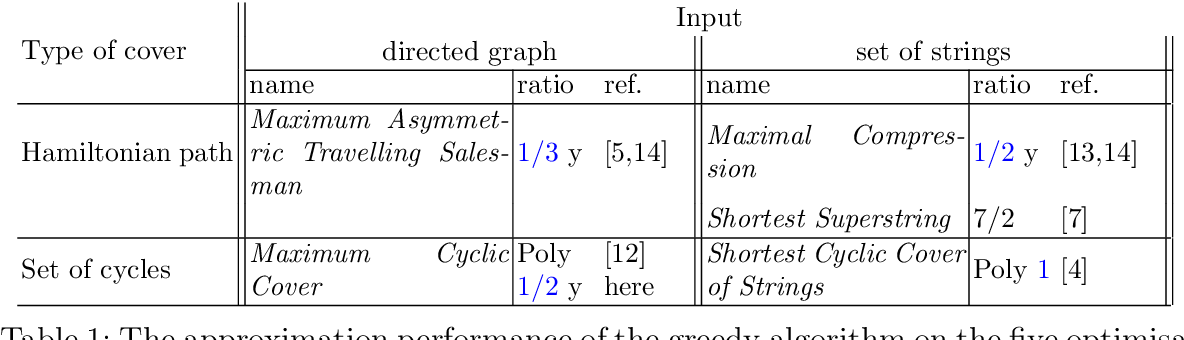•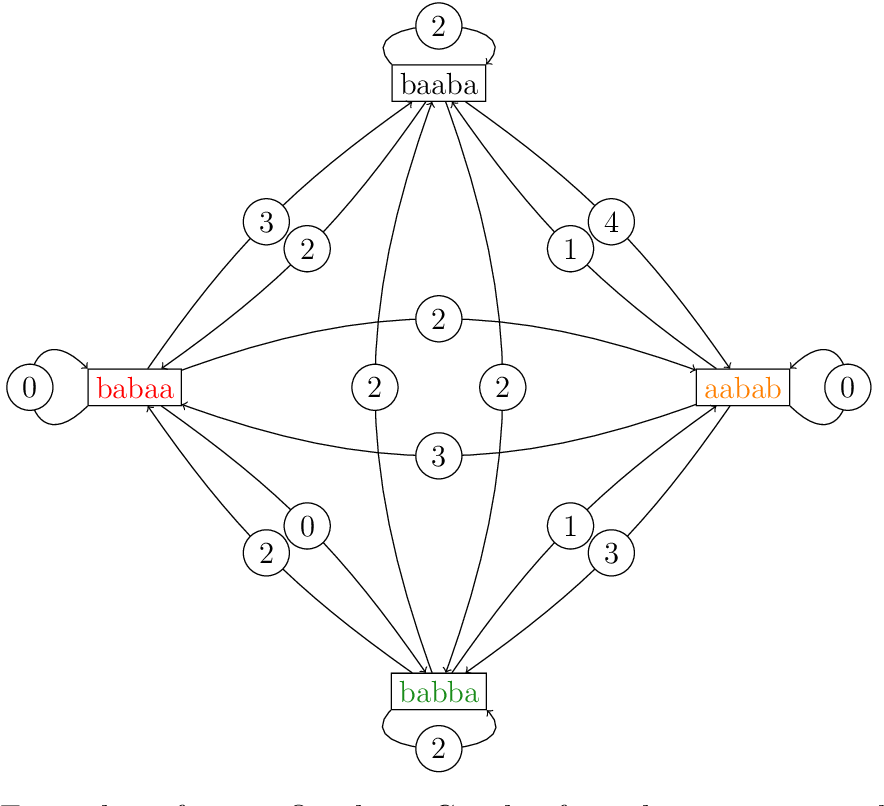•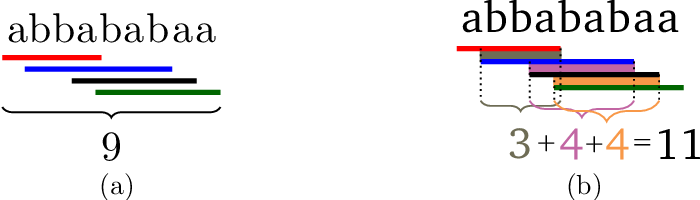•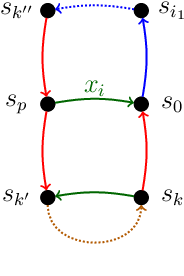2011
2011
• PRELUDE Project
• 2011
• Corpus ID: 6010348
In this paper, we use Girard's ludics to analyze focalization, a fundamental property of the proof theory of linear logic. In… Expand
2005
2005
• SIAM J. Sci. Comput.
• 2005
• Corpus ID: 25872246
Wavelet-based sparse approximate inverse preconditioners are considered for the linear system Ax=b. The preconditioners are good… Expand
•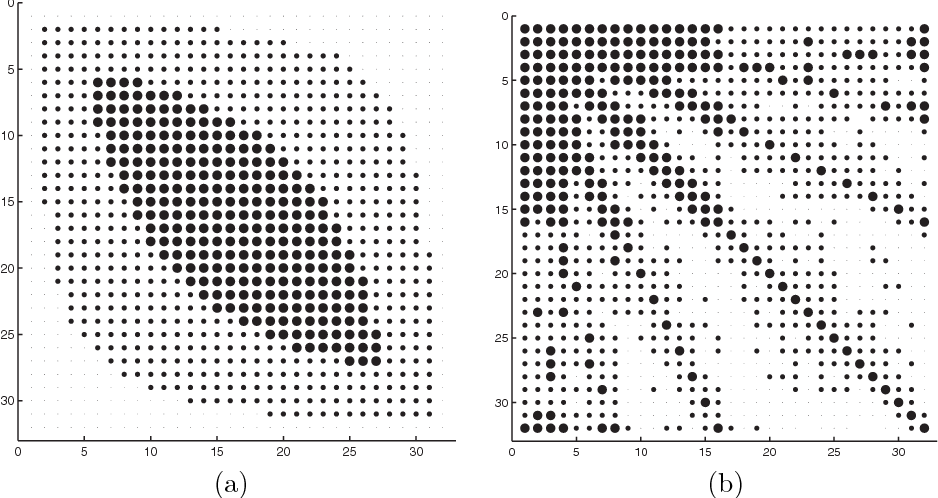•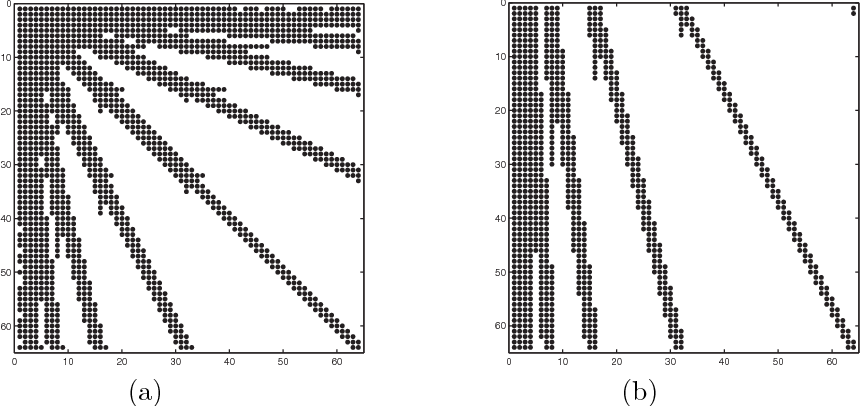•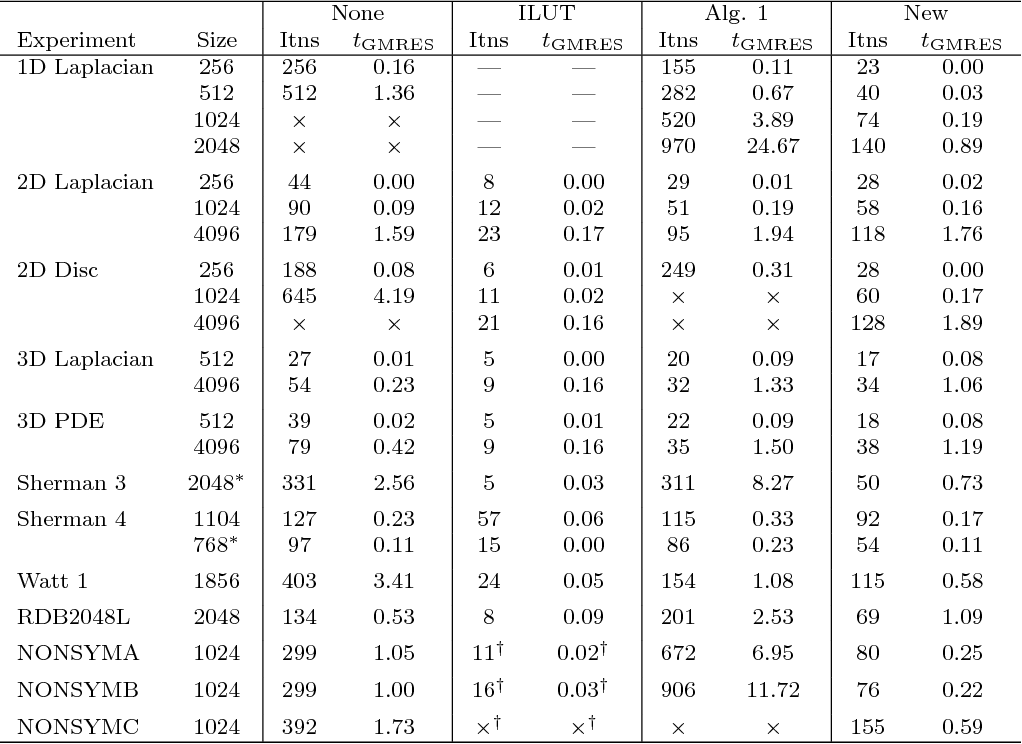•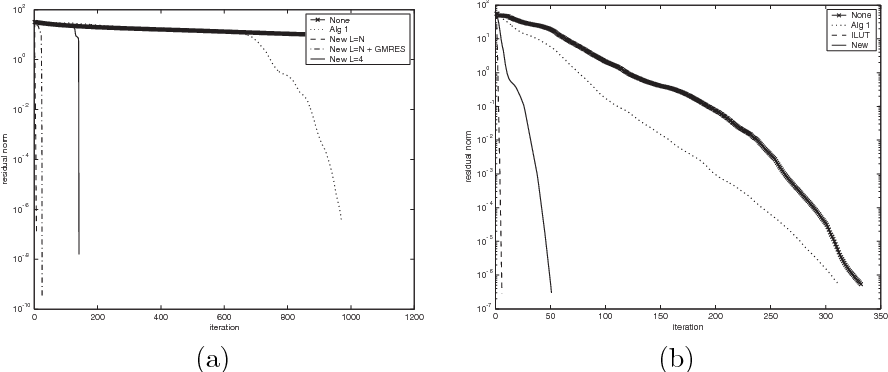•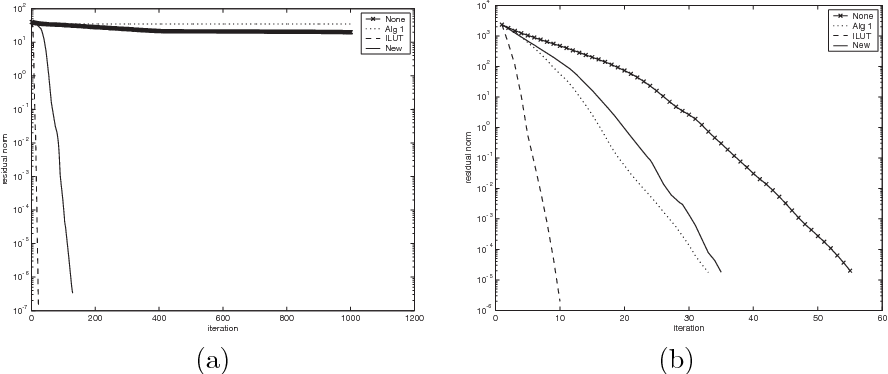Highly Cited
2003
Highly Cited
2003
• ICALP
• 2003
• Corpus ID: 687265
We prove lower bounds for the direct sum problem for two-party bounded error randomised multiple-round communication protocols… Expand
2002
2002
• T. Murayama
• Proceedings IEEE International Symposium on…
• 2002
• Corpus ID: 121895035
We analyze the performance of a linear code used for data compression of a Slepian-Wolf type. In our framework, two correlated… Expand
2001
2001
We analyze the performance of a linear code used for a data compression of Slepian-Wolf type. In our framework, two correlated… Expand
•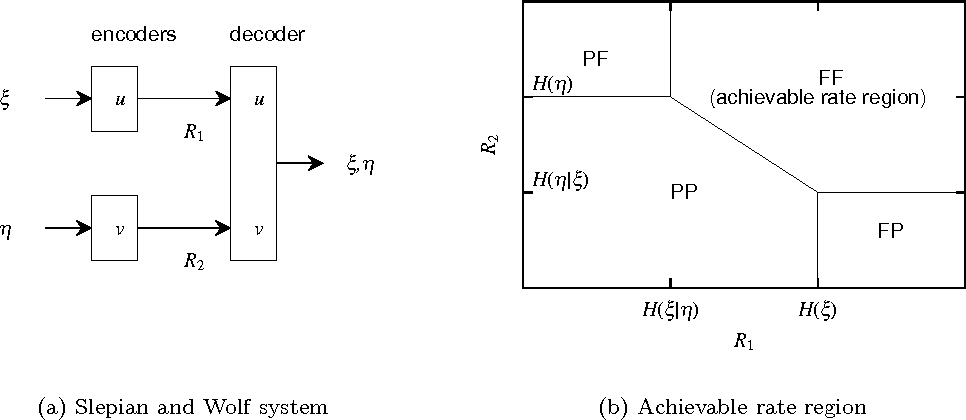•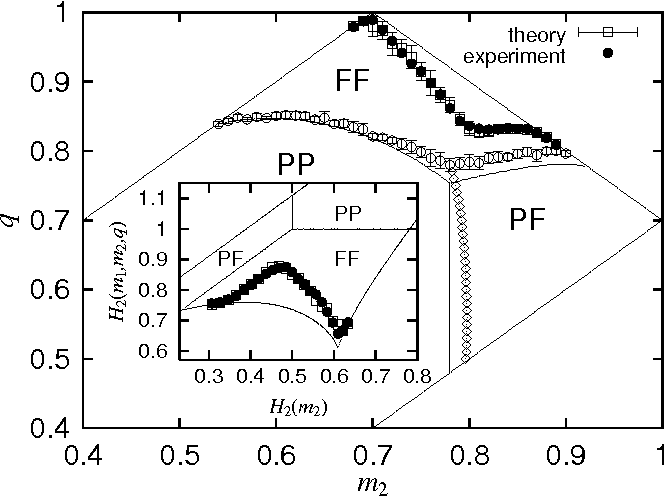2000
2000
• Discret. Math.
• 2000
• Corpus ID: 30364405
Suppose aibici(i=1,2) are two triangles of equal side lengths and lying on spheres Φi with radii r1,r2(r1<r2), respectively. We… Expand
1997
1997
• J. Comb. Optim.
• 1997
• Corpus ID: 35145869
AbstractSuppose AiBiCi (i = 1, 2) are two triangles of equal side lengths lying on spheres Φi with radii r1, r2 (r1 < r2… Expand
1994
1994
• Carl H. Smith
• Graduate Texts in Computer Science
• 1994
• Corpus ID: 206656265
From the Publisher: The aim of this textbook is to present an account of the theory of computation. After introducing the… Expand
Highly Cited
1975
Highly Cited
1975
• T. Cover
• IEEE Trans. Inf. Theory
• 1975
• Corpus ID: 7177935
If \{(X_i, Y_i)\}_{i=1}^{\infty} is a sequence of independent identically distributed discrete random pairs with (X_i, Y_i) ~ p(x… Expand